Next: Extinction efficiencies: general behaviour Up: Optical properties of cosmic Previous: Optical properties of cosmic

It is now evident that observed interstellar extinction cannot be explain using particles of only one kind. Therefore, three tendencies in the modelling of multi-component dust mixtures are being developed.

1. Several populations of compact (usually spherical) grains are used. Populations consist of different materials. The optical properties are calculated from the Mie theory. Such an approach was used many times for the interpretation of the interstellar extinction curves (e.g., Mathis et al., ; Zubko et al., ) and the SEDs in spectra of stars and YSOs (see Table 7 in Voshchinnikov ). In the case of circumstellar shells, the authors do not worry about the problem of cosmic abundances and can apply multi-component mixtures with varying fractional abundances at different distances from an object. For example, Men'shchikov et al. () chose a four-component mixture to explain observations of the young star HL Tau. The disadvantage of such an approach is the impossibility of including the vacuum as a component.

2. Several materials are mixed using one of the EMT's rules and then the Mie theory is applied for calculations of the optical properties of such composite'' particles. In this case, there exist many doubts on the validity of employing the EMT for significant fractional abundances of components as is discussed in Sect. 2.1.4 in Voshchinnikov .

3. Inhomogeneous (composite) particles with layers or inclusions from different materials or aggregate particles are considered and light scattering computations are made using the DDA, TMM or simpler theory like the Mie theory for-layered spheres. This approach seems to be the most promising because it allows one to describe more exactly the properties of dust grains. However, calculations with the DDA are very time-consuming and at the present can be used rather for illustrative than for mass calculations (e.g., Wolff et al., , Vaidya et al., ). The idea of composite particles as multi-layered spheres (Voshchinnikov and Mathis, ; see also Iatì et al., ) looks a bit artificial but attractive from the point of view of numerical realization (see discussion in Sect. 1.1).

The intensity of radiation after passing a dust cloudis equal to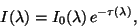(1)

where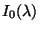is the source (star) intensity and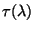the optical thickness along the line of sight. The interstellar extinction is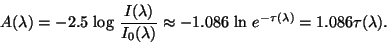(2)

Here,is the total extinction cross-section of all type particles along the line of sight in given direction.

For spherical particles of radius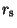, we have(3)

whereandare the column and number densities of dust grains, correspondingly andis the distance to the star.

For a polydisperse ensemble, averaging over a size distribution should be performed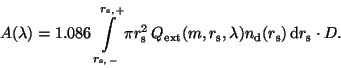(4)

Here,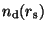is the size distribution of dust grains with the lower cut-off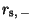and the upper cut-off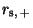.

From Eqs. (3) and (4), the important conclusion follows: the wavelength dependence of interstellar extinction is totally determined by the wavelength dependence of the extinction efficiencies.

SubsectionsNext: Extinction efficiencies: general behaviour Up: Optical properties of cosmic Previous: Optical properties of cosmic
root 2003-04-09